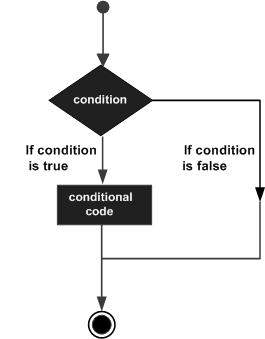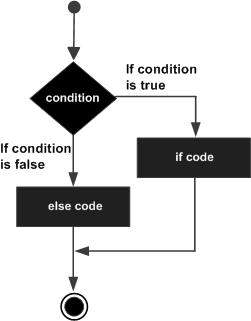# Explain this bit of code

This is for a temperature sensor. I want to understand what is gong on. Overall I think it is working as a threshold to only send a reading if it has changed at least certain amount, but I don't understand checkbound with 3 arguments or what the "return !isnan" does. No such thing as isnan previously declared anywhere.

``````bool checkBound(float newValue, float prevValue, float maxDiff) {
return !isnan(newValue) &&
(newValue < prevValue - maxDiff || newValue > prevValue + maxDiff);
}
``````

so it's the logic AND of

is this really a number

AND

Is the newValue outside maxDiff distance of the previous one

so it tells you basically if you have a valid input not something shooting through the rough or the cellar quickly

Okay, so it's not if it has changed at least some amount, but if the change was at most some amount.

What is the point of !isnan?
"is not not a number" which "is a number"?

Just dawned on me that it's a function declaration that you feed 3 values into. So it just returns a true/false due to bool?

How does that work with the 'if (checkbounds(x, y, z)){ do stuff}'
Does 'if' automatically not do anything if the condition is false?
It reads to me like "if (true) {do stuff}" but what keeps it from "if (false) {do stuff}" indifferently?

Float numbers can be "not a number"IEEE 754 NaNs are represented with the exponent field filled with ones (like infinity values), and some non-zero number in the significand (to make them distinct from infinity values); this representation allows the definition of multiple distinct NaN values, depending on which bits are set in the significand, but also on the value of the leading sign bit (not all applications are required to provide distinct semantics for those distinct NaN values).

Let's say you do some maths and you have an error (distinct from infinity) Then the NaN values are used to identify undefined or non-representable values for floating-point elements, such as the square root of negative numbers or the result of 0/0.

Yes it returns basically something that says "is the last reading I got correct"? - which when you follow a slow evolving process through some sensors means that nothing bad is happening and if your reading is out of norm then you shut down the system or take corrective action

You could do indeed

if (checkbounds(x, y, z)) {} else {}

``````if (checkbounds(x, y, z)){ do stuff}
``````

is the same as

``````if (checkbounds(x, y, z) == true){ do stuff}
``````

Not sure if that makes it clearerJ-M-L:
Is the newValue within maxDiff distance of the previous one
...
not something shooting through the rough or the cellar quickly

The comparison is "without" not "within". It is a hysteresis check not a bounds check.

I noticed that too and actually corrected my first post at 6:23 while you were probably typing as you posted at 6:26...

Sorry for that

sterretje:

``````if (checkbounds(x, y, z)){ do stuff}
``````

is the same as

``````if (checkbounds(x, y, z) == true){ do stuff}
``````

Not sure if that makes it clearerThat helps clarify what I meant when I was asking.

The code only has

``````if (checkbounds (x, y, z)){ do stuff}
``````

There is no else, there are no other ifs. Just wondering if the 'if' cares about TRUE without needing to be explicit as the second thing you put,

There is no else, there are no other ifs. Just wondering if the 'if' cares about TRUE without needing to be explicit as the second thing you put,

well IF cares about an expression that evaluates to 0 or non 0. if it's 0 then it considers false and will go to the else clause if there is one or just jump to the next instruction after the if without entering the IF {do stuff} statement.

that's why you can do if (digitalRead(12)) { } and you don't need to do if (digitalRead(12) == HIGH) { } . The first version is considered poor programming practice though because you test something that is not really a LOGIC value (but you know how things work and thus that will not make your program crash and burn..). Some programming language or compiler would actually enforce that you use a logic element in test to prevent errors

When you do (x == y) that's an expression that gets evaluated and returns 1 (true) if x equals y or 0 (false) if not and this is conceptually a LOGIC value you get back. So it feels good to have that in a if clause.

so if (checkbounds(x, y, z)) {} is all correct because what is in the IF is a LOGICAL value (because the function returns a boolean) but if (checkbounds(x, y, z) == true) is also correct and will check if the LOGIC value returned by the fonction is equals to the LOGIC value true and return the logic evaluation

makes sense?

from www.tutorialspoint.com as a picture is worth 1000 wordsYeah makes sense. Just not seeing the == in the 'if' bubble threw me off. I read it as "if the checkbounds function returns anything" and I wasn't sure what it was returning for what conditions.